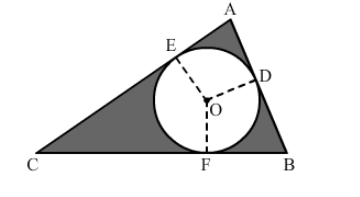# In the given figure, ∆ABC is right-angled at A.`
Question:

In the given figure, ∆ABC is right-angled at A. Find the area of the shaded region if AB = 6 cm, BC = 10 cm and O is the centre of the incircle of ∆ABC.Solution:

Using Pythagoras' theorem for triangle ABC, we have: